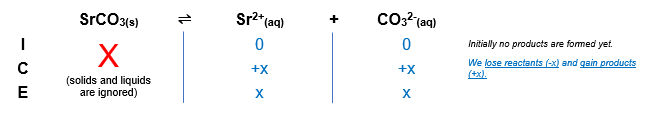# Problem: Find the molar solubility of SrCO3 (Ksp = 5.4 x 10-4) in (a) pure water and (b) 0.13 M Sr(NO3)2

###### FREE Expert Solution

The dissociation of SrCO3 in water is as follows:

SrCO3(s) ⇌ Sr2+(aq) + CO32(aq)

(a) Pure water

We can construct an ICE table for the dissociation of SrCO3

Remember that solids are ignored in the ICE table and Ksp expression.$\overline{){{\mathbf{K}}}_{{\mathbf{sp}}}{\mathbf{=}}\frac{\mathbf{products}}{\overline{)\mathbf{reactants}}}{\mathbf{=}}{\mathbf{\left[}}{{\mathbf{Sr}}}^{\mathbf{2}\mathbf{+}}{\mathbf{\right]}}{\mathbf{\left[}}{{{\mathbf{CO}}}_{{\mathbf{3}}}}^{{\mathbf{2}}{\mathbf{-}}}{\mathbf{\right]}}}$

92% (152 ratings)###### Problem Details

Find the molar solubility of SrCO3 (Ksp = 5.4 x 10-4) in (a) pure water and (b) 0.13 M Sr(NO3)2